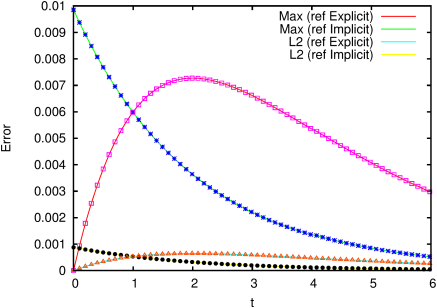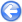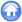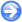### 14.2PASS: Relaxation of a charge bump

Author
Jose M. López-Herrera Sánchez
Command
sh bump.sh
Version
100610
Required files
bump.sh norms-explicit.ref norms-implicit.ref
Running time
1 minutes 14 seconds

A concentrated bump of charge of the form,

ρe(x,t=0)=
exp
 x2+y2 2a2
a
 2 π

is initially set in a single fluid of conductivity K and permittivity ε. Due to conduction the bump decays exponentially in time (see figure 200) as

 ρe(x,t)=ρe(x,t=0) exp(−K t/ε)

while preserving the initial geometry (see figure 201).

A more detailed discussion of this simulation is given in .

 Figure 200: Temporal decay of the charge density at (0,0). (Continuous) analytical solution, (symbols) Gerris EHD module.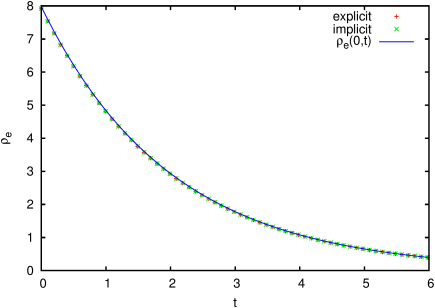Figure 201: Spatial distribution of charge density along the radial axis (times equal 0, 2, 4 and 6 are shown). (continuous) analytical solution, (symbols) present simulation.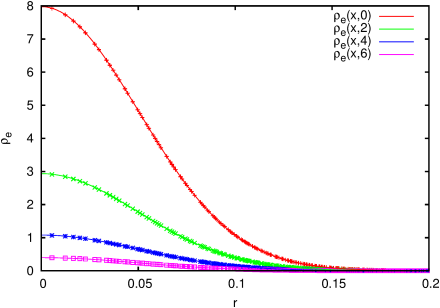Figure 202: Evolution of the norms of the error on the charge density distribution shown in figure 201.